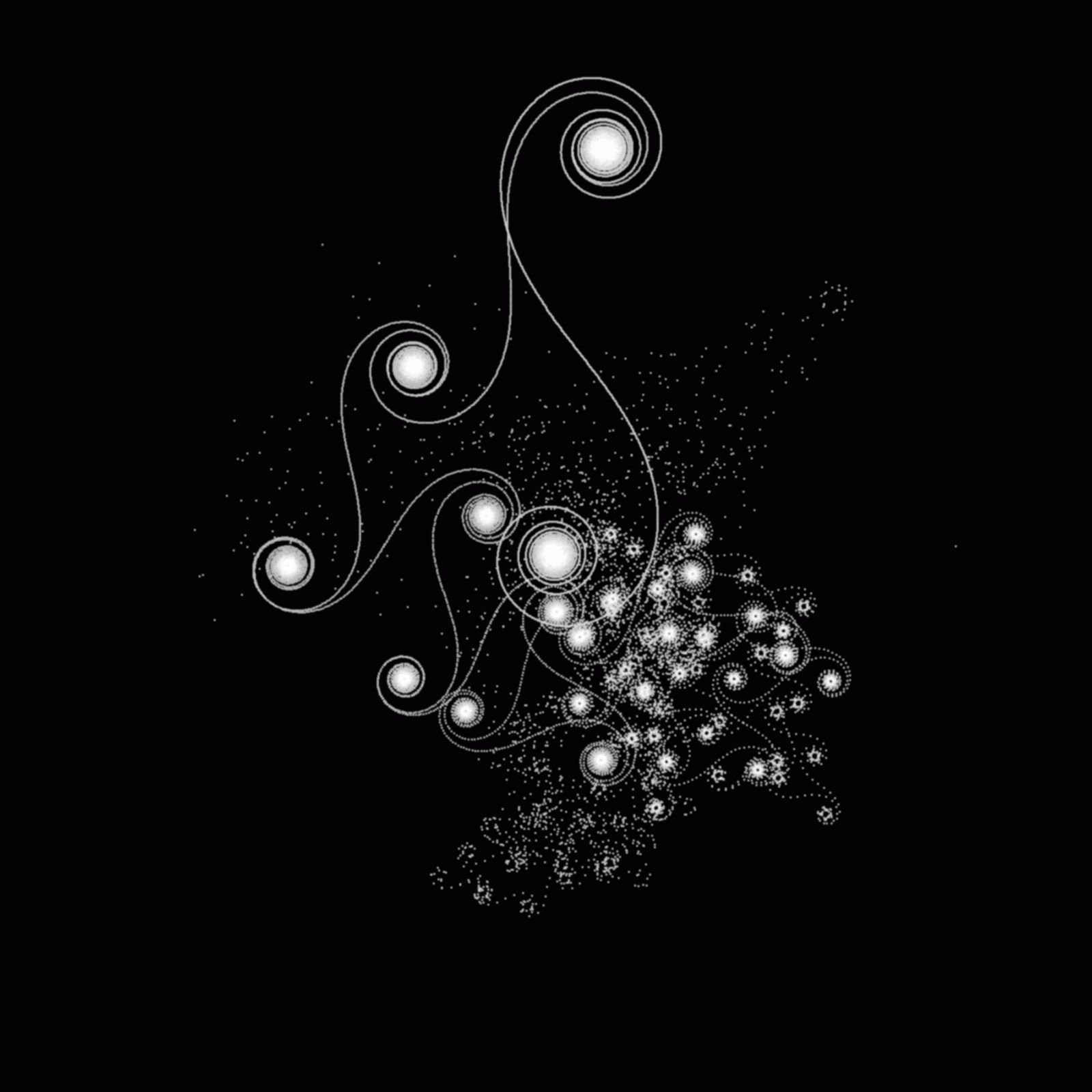# A Graphical Analysis of the Riemann Zeta FunctionA Graphical Analysis of the Riemann Zeta Function is a paper revealing and explaining some of the
aesthetically and mathematically interesting visual patterns
found in the Zeta function by graphing the first n terms of the function on the complex plane.

Graphs

Graph 1 (s = 1/2 + 936391i)
Graph 2 (s = 1/2 936391i)
Graph 3 (s = 1/2 + 210,000,000,000.000i)
Graph 4 (s = 1/2 + 267,653,395,648.848i)
Graph 5 (s = 1/2 + 267,653,395,648.000i)

Images of the Zeta Function viewed as a Terrain Height Map

zetaland (zipped)

Animations of the Zeta Function

Animation 1 (s = 1/2 + 936389i)
Animation 2 (s = 1/2 + 2.10,000,000,000.000)

C Code

zeta3.txt
zeta3_1.txt

Home

Email - [email protected]

Copyright(C) 2005 Max Radin
Hosted by www.Geocities.ws